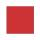# Perimeter of square

The square has a circumference 17cm. What is its area?

Result

S =  18.063 cm2

#### Solution:Leave us a comment of example and its solution (i.e. if it is still somewhat unclear...):

Showing 0 comments:Be the first to comment!## Next similar examples:

1. Perimeter from areaWhat is the perimeter of the square if its content is 64 cm2?
2. Area of squareCalculate the content area of the square whose perimeter is 24 dm.
3. Circle - simpleThe circumference of a circle is 930 mm. How long in mm is its diameter?
4. Bicycle wheelAfter driving 157 m bicycle wheel rotates 100 times. What is the radius of the wheel in cm?
5. Circle - easy 2The circle has a radius 6 cm. Calculate:
6. RectangleThe rectangle area is 182 dm2, its base is 14 dm. How long is the other side? Calculate its perimeter.
7. Clock handsThe second hand has a length of 1.5 cm. How long does the endpoint of this hand travel in one day?
8. Square prismA square prism has a base with a length of 23 centimeters, what is the area in square centimeters of the base of the prism?
9. 3x squareSide length of the square is 54 cm. How many times increases the content area of square if the length of side increase three times?
10. Dropped sheetsThree consecutive sheets dropped from the book. The sum of the numbers on the pages of the dropped sheets is 273. What number has the last page of the dropped sheets?
11. Negative in equation2x + 3 + 7x = – 24, what is the value of x?
12. 22/7 circleCalculate approximately area of a circle with radius 20 cm. When calculating π use 22/7.
13. AnnulusThe radius of the larger circle is 8cm, the radius of smaller is 5cm. Calculate the contents of the annulus.
14. CableCable consists of 8 strands, each strand consists of 12 wires with diameter d = 0.5 mm. Calculate the cross-section of the cable.
15. HotelThe hotel has a p floors each floor has i rooms from which the third are single and the others are double. Represents the number of beds in hotel.
16. RoundingThe following numbers round to the thousandth:
17. AlleyAlley measured a meters. At the beginning and end are planted poplar. How many we must plant poplars to get the distance between the poplars 15 meters?